# Joint Probability

• Updated on

What is meant by joint probability?

Joint probability, in probability theory, refers to the probability of two events occurring together.  The formula for estimating joint probability is as follows:-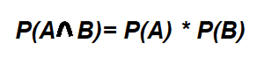Here, A and B are two independent events. P(A) is the probability of event A, and P(B) is the probability of the occurrence of event B. Thus we see that the probability of both event A and event B happening is the product of the probability of their independent occurrence. The occurrence of each event mustn't be dependent on the occurrence of the other.

The joint probability estimation for two events can be represented using a Venn diagram. For example, the probability of getting two heads on tossing two fair coins can be shown as follows: -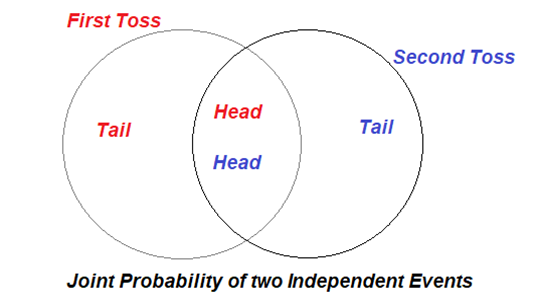Summary
• Joint probability is a concept in probability theory that refers to the probability of two events occurring together.
• Two events are said to be independent if knowing one event occurred does not affect the likelihood of the other event.

What is probability theory?

A field of mathematics concerned with the examination of random processes is known as probability theory. A random event's outcome cannot be predicted before it occurs, although it could be any of the multiple possibilities. The final result is said to be dictated by chance.

We are surrounded by randomness. Probability theory is a mathematical framework that allows us to understand chance events using rationale and a systematic approach. The probability of an occurrence is a number that indicates how probable it is to happen. This number is always in the range of 0 to 1, with 0 denoting impossibility and one denoting certainty.

Few examples of chance events are getting a tail on tossing a coin, getting a sum greater than ten on tossing two coins, rains two days in a row, drawing an ace card from a pack of cards, guessing a lottery number, getting accurate results from an experiment etc. Various principles of probability theory are employed to examine the likelihood of the occurrence of such events.

What are independent events in probability theory?

Two events are said to be independent if knowing one event occurred does not affect the likelihood of the other event. For example, if a die is rolled once each day, then the probability of getting a six on rolling a die once will be regardless of which day of the week it is. So if we consider one event to be rolling a die once each day and getting a six and the other event to be the day being Friday, then these two are said to be independent events because regardless of the day, when a dice is rolled once, the probability of getting a six will be 1/6 only.

If there are two events- event A and event B, they are said to be independent if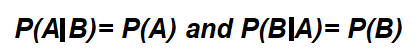Where P(A|B)= probability that event A will occur if event B has occurred and

P(B|A)= probability that event B will occur if event A has occurred.

In the example given above, event A= Getting a six on rolling a die once on a day, and P(A)=1/6

Event B= The day is Friday, and P(B)= 1/7

P(A|B)= 1/6 and P(B|A)=1/7

So the joint probability in this case is a situation of throwing a dice once on a Friday and getting a six. The probability of such an event will be P(A)*P(B)= (1/6)*(1/7)= 1/42

What is the difference between joint probability and conditional probability?

The likelihood of an event occurring if another event has already occurred is known as conditional probability. However, in the case of conditional probability valuation,  a causal relationship or simultaneous occurrence of the events is not a must. The formula for conditional probability is given as: -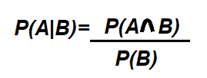P(A|B) is the conditional probability of event A occurring given that event B has already occurred, P (A ∩ B) is the joint probability of events A and B- the probability that both events A and B occur, and P(B) is the probability of event B. If events A and B are not independent, then the probability of the intersection of A and B is defined by P(A and B) = P(A)*P(B|A). This helps us arrive at the above formula.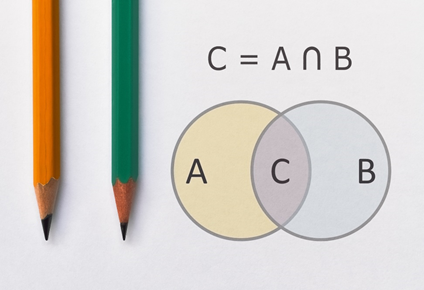Intersection Of Two Sets© Benjaminec | Megapixl.com

This is different from the joint probability formula, which says P(A|B) = P(A)- this is the difference because P(A) will not change regardless because events A and B are independent.

Opposingly, if the scenario is that events A and B are mutually exclusive, i.e. if one has occurred and the other cannot occur. For example, there is a bag with three balls- red, green and blue, and two balls are drawn without replacement. Event A is that the first ball is red, and event B is that the second ball is red. Here we see that since there is only one red ball and thus events A and B cannot occur together. Thus P(A|B)= 0 (because the probability of A and B occurring together is zero) while P(A)= 1/3.

How is joint probability estimated?

Joint probability estimation can be understood using the following examples-

Suppose there are two bags of masks, and one mask is selected randomly from each bag. One bag has ten white masks, and the other has ten black masks. What will be the probability of choosing one white and one black mask?

Here we see that since the masks are picked from different bags, both are independent events.

P (choosing a white mask) = 1/10

P (choosing a black mask) = 1/10

P (choosing one white and one black mask) = (1/10) *(1/10) = 1/100

However, if the situation were to find the probability of picking one black and one white mask from a bag of 20 red and white masks with ten of each colour, then the conditional probability would have to be applied.

We use cookies to ensure that we give you the best experience on our website. If you continue to use this site we will assume that you are happy with it. OK